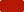Track It for Life and Stay Healthy for Life

The Harris Benedict Equation is a formula that uses your BMR (Basal Metabolic Rate) and then applies an activity factor to determine your total daily energy expenditure (calories). The only factor omitted by the Harris Benedict Equation is lean body mass. Remember, leaner bodies need more calories than less leaner ones. Therefore, this equation will be very accurate in all but the very muscular (will under-estimate calorie needs) and the very fat (will over-estimate calorie needs).

Body Mass Index (BMI) describes relative weight for height and is signifi cantly correlated with total body fat content.

 Gender: FemaleMale Height: 4'5'6'7'   0"1"2"3"4"5"6"7"8"9"10"11" Age: Weight: (pounds)

BMI Categories:
• Underweight = <18.5
• Normal weight = 18.5-24.9
• Overweight = 25-29.9
• Obesity = BMI of 30 or greater

 Activity Factor Total Daily Calorie Needs (KCal/Day) Calorie Goal to Lose 1 Lb Per Week 1. Sedentary: Multiply BMR by 1.2 KCal per Day KCal per Day 2. Lightly Active: Multiply BMR by 1.375 KCal per Day KCal per Day 3. Moderately Active: Multiply BMR by 1.55 KCal per Day KCal per Day 4. Very Active: Multiply BMR by 1.725 KCal per Day KCal per Day 5. Extra Active: Multiply BMR by 1.9 KCal per Day KCal per Day

To determine your total daily calorie needs, multiply your BMR by the appropriate activity factor, as follows:

1. If you are sedentary (little or no exercise)
Calorie-Calculation = BMR x 1.2
2. If you are lightly active (light exercise/sports 1-3 days/week) :
Calorie-Calculation = BMR x 1.375
3. If you are moderatetely active (moderate exercise/sports 3-5 days/week)
Calorie-Calculation = BMR x 1.55
4. If you are very active (hard exercise/sports 6-7 days a week)
Calorie-Calculation = BMR x 1.725
5. If you are extra active (very hard exercise/sports & physical job or 2x training) :
Calorie-Calculation = BMR x 1.9